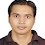Find S Algorithm with Example - JOB SAARNEE

# Find –S Algorithm In Find –S algorithm we tend to find a Maximally Specific Hypothesis that fits the all positive training examplesSome important points related to Find –S algorithm: 1. Find-S algorithm only considers positive training examples and neglect negative training examples. 2. In Find-S algorithm, we move from top to bottom i.e. specific hypothesis to general hypothesis. In the other words we can say that in Find-S algorithm we start with the most specific hypothesis and generalizes this hypothesis each time whenever the attributes values of hypothesis and attributes values of observed positive training example did not match. 3. Maximally specific hypothesis A hypothesis h, is a maximally specific hypothesis if it covers none of the negative training examples and there is no other hypothesis h’ that covers none negative training examples, such that h is strictly more general than h’. Notations used in Find-s algorithm: 1. The most specific hypothesis is represented by the by the {φ,φ,φ,φ} where number of the ‘φ’ is equal to number of attributes in training data. 2. ‘φ’ indicate that no value is acceptable for the attributes. 3. ‘?’ Indicate that any value can be acceptable for the attributes. Find –s algorithm: Step 1: Initialize h to most specific hypothesis h. Step 2: For each positive training instance x Step 3: for each attribute’s constraint ai in h                if the constraint ai is satisfied by x                   Then does nothing                 else                replace ai in h by the next general hypotheses                 Constraint ‘?’ that is satisfied by x. Step 4: Output hypothesis. Example: - To understand this algorithm, we consider the below training example. Outlook Temperature Humidity Wind Play tennis Overcast Rain Rain overcast Hot Mild Cool Cool High High Normal Normal Weak Weak Strong Weak Yes Yes No yes In the above training example, target attributes are play Tennis. First, we initialize h to most specific hypothesis: h = {φ, φ, φ, φ} Now we consider first training example: x1 = (Overcast, Hot, High, Weak) This is the positive training example.  From here, it is clear that none of the attributes value in h is satisfied with the attributes value in x1. So, each attribute in h is replaced by the next general constraints – h1 = (Overcast, Hot, High, Weak) Now, we consider second training example: x2 = (Rain, Mild, High, Weak) This is positive training example. We compare each attribute value in h1 with the attributes value in x2 and substitute ‘?’ in the place of any attributes value in h if it is not satisfied with x2. h2 = (‘?’, ‘?’, High, Weak) Now, we consider third training example: x3 = (Rain, Cold, Normal, Normal) This is negative training example. so, we neglect this training example and proceed further. Now we consider fourth training example. x4 = (Overcast, Cool, Normal, Weak) This is positive training example. After comprising each attribute value in h2 with the attributes value in x4,  we have- h3 = (‘?’, ‘?’, ‘?’, weak) This is the output hypothesis.

1.2.